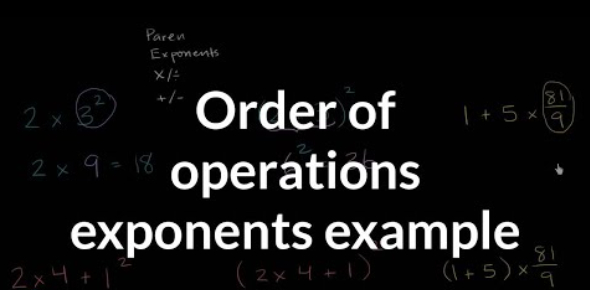# Operations With Exponents Quiz! Math Trivia

8 Questions | Total Attempts: 52Settings. Operations with exponents quiz math trivia. There are a lot of people who have a hard time-solving math problem involving exponential numbers. For you to know what to do when it comes to solving such problems, you need to understand rules for working out different functions. By taking this quiz, you will be able to get some much-needed practice. Do give it a shot and see how well you do!

• 1.
Re-write in a simpler form:
• A.
• B.
• C.
• D.
• 2.
Re-write in a simplier form:
• A.
• B.
• C.
• D.
• 3.
Re-write in a simpler form: 8+8+8+8
• A.
• B.

8 x 4

• C.
• D.

There isn't a simpler form

• 4.
Simplify completely.
• A.

64

• B.

16

• C.

32

• D.

10

• 5.
Simplify completely:
• A.

3

• B.

6

• C.

9

• D.

12

• 6.
Simplify completely:
• A.

1

• B.

23

• C.

5

• D.

31

• 7.
Simplify completely:
• A.

0

• B.

16

• C.

1

• D.

2

• 8.
Simplify completely:
• A.

30

• B.

72

• C.

36

• D.

24

Related TopicsBack to top